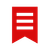# QlikView App Dev

Discussion Board for collaboration related to QlikView App Development.

Announcements
Support Cases coming to Qlik Community Oct. 4! Start chats, open cases, explore resources: READ DETAILS
cancel
Showing results for
Did you mean:Master II

## Multiple IF Condition

Hi,

I've been trying to implement multiple IF condition.

I've achieved it when I had 2 if's now I need to add 3rd if which can also be done but the problem is it's range based.

Ex:

if(\$(v123)>=1000000,'A',

if(\$(v123)<=100000,'B'))

Now I want the result if 123 is >1000000 as A

If 123 is < 100000 as B

and if 123 is in between 1 and 99999 as C

Basically it is in between a particular number

Ex:between 1 to 100 is A, between 100 to 1000 is B and greater than or equal to 10000 is C

Thanks.

@

1 Solution

Accepted SolutionsMVP

Here you go....I added a fourth condition where if the number is less than 1000, it would be like '\$#,##0.0'

Expression:

=If(Sales >= 1000000000, Num(Sales/1000000000, '\$#,##0.0 B'),

If(Sales >= 1000000, Num(Sales/1000000, '\$#,##0.0 M'),

If(Sales >= 1000, Num(Sales/1000, '\$#,##0.0 K'), Num(Sales, '\$#,##0.0'))))

27 RepliesMVP

May be this:

if(\$(v123)>=1000000,'A',

if(\$(v123)<=100000,'B', 'C'))MVP

Or if you want to be explicit, then this

If(\$(v123) <= 100000, 'B',

If(\$(v123) < 1000000, 'C',

If(\$(v123) >= 1000000, 'A')))Champion II

Like below

if(123 <= 100,'A',if(123 < 1000,'B','C'))Master II
Author

I already tried all these conditions but doesn't seem to give me 3rd result.

Just giving A & B

I'm using this in text box

I want to show a particular number to be in B if >10000000

if <10000000 in M

And then I also want to show in K

I'm using

if(\$(expressionstoredinVariable)>1000000000, num(\$(expressionstoredinVariable)/1000000000, '\$#,##0.00 B' and so.....Master II
Author

My current expression which is not working for K

=if(\$(Variable)>=1000000000 ,num(\$(Variable)/1000000000, '\$#,##0.00 B'),

if(\$(Variable)<=10000000000 ,num(\$(Variable)/10000000, '\$#,##0.00 M'),

if(\$(Variable)<1000000000 ,num(\$(Variable)/10000000, '\$#,##0.00 K'))))

The problem here is it will show in M only cus we taking <10000000 as M and everything will be less after that, hence will be shown in M.Master II
Author

Tried but n/wMaster II

Hi,

=If(Sales>1 and Sales<=100,'A',if(Sales>100  and Sales<=1000,'B','C'))MVP

May be this

=if(\$(Variable) >= 1000000000,

num(\$(Variable)/1000000000, '\$#,##0.00 B'),

if(\$(Variable) >= 10000000,

num(\$(Variable)/1000000, '\$#,##0.00 M'),

num(\$(Variable)/1000, '\$#,##0.00 K')))Partner

=if(\$(Variable)<1000000000 ,

num(\$(Variable)/10000000, '\$#,##0.00 K'),

if(\$(Variable)<=10000000000,

num(\$(Variable)/10000000, '\$#,##0.00 M'),

if(\$(Variable)>=10000000000,

num(\$(Variable)/1000000000, '\$#,##0.00 B')

)

)

)Community Browser